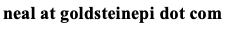# Neal D. Goldstein, PhD, MBI

Nov 19, 2015

### The why and when of centering continuous predictors in regression modeling

The notion of centering and scaling continuous variables in regression is the source of constant debate and questioning (Example #1, Example #2) and often answers are given in terms statistical properties. For the epidemiologist, what are the practical implications? In other words, when should a continuous variable be centered (and/or standardized) before running the regression model? Centering a variable moves its mean to 0 (which is done by subtracting the mean from the variable), standardizing adjusts the scales of magnitude (by dividing the centered variable by its standard deviation). There are actually only a few instances when either is appropriate in typical epidemiological studies, and you will generally recognize when its necessary by model convergence issues and/or inflated standard errors. Here's a concise list, recognizing that one or more may apply at any given time:

1. Interpreting the intercept. If the intercept term needs to be interpreted, and any predictor variable does not have a meaningful 0 value (such as weight or height) then the predictor should be centered.
2. Interactions. If you are testing an interaction between a continuous variable and another variable (continuous or categorical) the continuous variable(s) should be centered to avoid multicollinearity issues, which could affect model convergence and/or inflate the standard errors. See this reference.
3. Polynomial terms. If you are transforming a variable (x^2), the transformed variable may be highly correlated with the untransformed variable (x). For the same reason as the interaction term, center the untransformed variable (x) after the transformation.
4. Multilevel analysis. Because intercept terms are of importance, it is often the necessary to center continuous variables. Additionally, the variables at different levels may be on wildly different scales, which necessitates centering and possibly scaling. If the model fails to converge, this is often the first check. See this discussion.
5. Coefficient interpretation. Occasionally when independent variables are on very different scales (e.g., age, income, population, all in the same model), the coefficients may not have meaningful values (for each dollar change is probably not of interest), therefore standardizing the units helps for interpretation.

Users of R can refer to the built-in scale() function, which allows both mean centering and standardization of a continuous variables. Other statistical software may have similar features, or require manually centering or scaling the variable(s).

Cite: Goldstein ND. The why and when of centering continuous predictors in regression modeling. Nov 19, 2015. DOI: 10.17918/goldsteinepi.